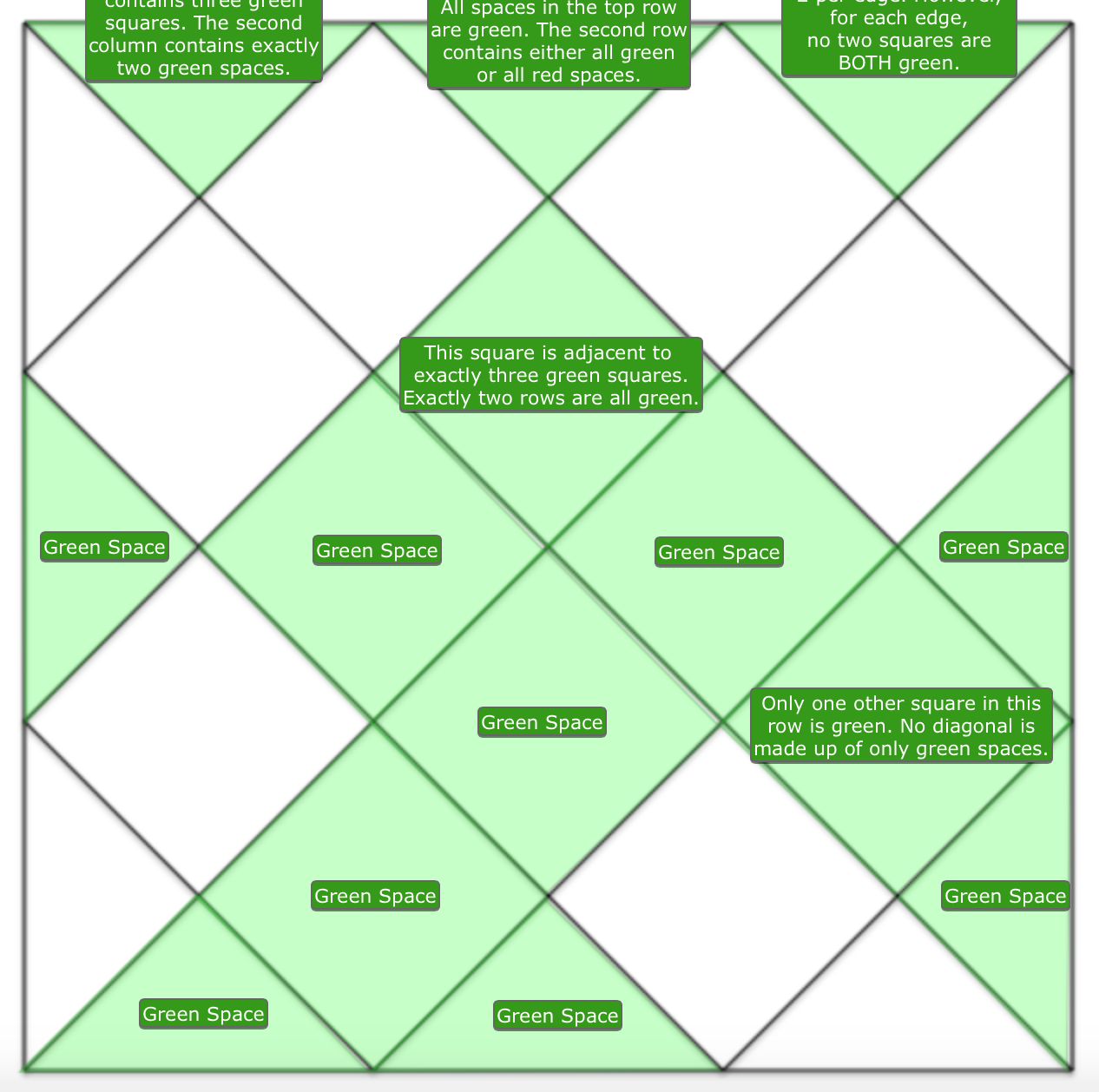1) The top-center triangle is GREEN, as indicated in the instructions.

2) That triangle indicates that the entire top row is green. So the two adjacent triangles are also GREEN.

3) The green triangle in the center says that the row below the green triangles is either all green or all red. However, the green triangle on the right says that the two squares that are on each edge of the grid are not both green. So the entire 2nd row must be RED.

4) The green triangle on the left shares a diagonal with three green squares. Since the one adjacent to it is red, then the remaining squares in the diagonal are GREEN.

5) The rightmost square in the 3rd row is RED because the other square along the right edge is green.

6) The bottom-right green square indicates that one other square in its row is green. Let’s pretend the green square is the left box in that row. Then the square above it must be red (satisfying the squares on edges rule). Additionally, the top-center square says that it is adjacent to three green squares. The second row squares and the third row square on the right are all red, so only two squares remain to be green. However, one of those squares in in the row that we are concerned about, so this scenario does NOT work. Thus, the center square in the 5th row is GREEN.

7) As a result, the left-most square in the 5th row is RED.

8) Let us do a little math here. We need 14 green spaces, and we discovered 7 so far. One of the left-most squares in the 3rd and 4th rows must be green (but not both) to satisfy three green squares adjacent to the center square in the third row. That makes 8 green spaces. We eliminated the entire 2nd row, the right square in row 3, and the left square in row 5. So we have 6 more green spaces in 9 possible locations. The bottom-right green square indicates that no entire diagonal is composed of only green spaces. So each set of two triangles in each corner must have at least one red triangle (both triangles make up a diagonal according to the definition in the “How to Play”). If we assume one triangle in each corner is red, there’s now 6 greens in 7 spots. Look at the two squares in the 6th row. They both can’t be green (because of squares next to edges rule), but they also both can’t be red because there is only room for one more red box. If we now assume that one of those two squares is red, we have six green spaces in six spots. The only spaces that do not contain uncertainty now are the center triangles on the edges of the grid. So those three triangles are GREEN.

9) The right square in the 6th row has to be red because that would make its diagonal completely green, violating the clue in the space right next to it. So that space is RED, and the space to the left of it is GREEN.

10) Three green boxes remain. The top-center square indicates that two rows are all green. The first row is already green, so one row remains. From previous instructions, the second row, third row, 5th row, and 6th row cannot be all green. That leaves the 4th and 7th rows as possible candidates. If the 7th row was all green (the row of triangles at the bottom), then that would satisfy two green spaces in the second column (a clue stated in the top row). That means the last green space would be in the 4th row, but that row would then also be green. As a result, the bottom row is not all green and instead, the 4th row is all green. So the remaining space in that row is GREEN, and the leftmost square in the third row is RED.

11) We still have to satisfy two green spaces in the second column. So the triangle at the bottom of that column is GREEN.

12) The remaining triangle in the bottom row must be red so that it does not make the entire row. As a result, the adjacent triangle in the bottom-right corner is GREEN.